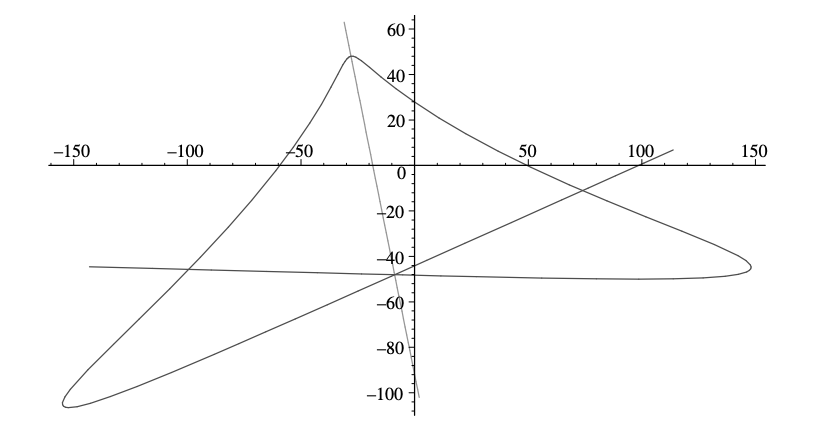시간 제한 메모리 제한 제출 정답 맞은 사람 정답 비율
2 초 512 MB 0 0 0 0.000%

## 문제

A symmetric polynomial is a polynomial in n variables that remains the same polynomial under any permutation of the variables. For example, f(x1, . . . , xn) = x1 + . . . + xn is a symmetric polynomial (it is in fact called the first elementary symmetric polynomial). Symmetric polynomials have many important applications. They can, for instance, be used to prove that there is no formula for the roots of a general five-degree polynomial.

In this problem however, we will concern ourselves with another kind of symmetry. Consider an infinite curve P in the plane where both x and y coordinates are given by polynomials, i.e.,

x(t) = antn + an−1tn−1 + . . . + a1t + a0,

y(t) = bmtm + bm−1tm−1 + . . . + b1t + b0.

We say that such a curve is symmetric around a straight line L (given as an equation Ax + By + C = 0) if there exists a real number t0 such that for all t ∈ R the point (x(t0 + t), y(t0 + t)) is the reflection of (x(t0 − t), y(t0 − t)) around the line L, and we call the line L a symmetry line for the curve P. For example, consider the curve P given by

x(t) = −5t5 − 26t4 − 19t3 + 59t2 + 111t + 26,

y(t) = −t5 + 17t3 − 9t2 − 61t + 12.

This curve is symmetric around the line 5x + y + 92 = 0 with t0 = −1 (see Figure G.1).Figure G.1: Illustration of Sample Input 1, drawn from t = −3.9 to t = 1.9.

Now, your task is to write a program that, given the two polynomials x(t) and y(t), finds a symmetry line of the curve (if one exists).

## 입력

The first line of input contains an integer n (0 ≤ n ≤ 10), the degree of x. Then follows a line with n + 1 integers an, . . . , a1, a0, where ai is the degree i coefficient of x. Then follow two lines describing the polynomial y in the same format.

If either of x(t) or y(t) is the zero polynomial, its degree is given as 0. The coefficients have absolute values bounded by 1 000. You may assume that the leading coefficient an of each polynomial is non-zero, except in the case when the polynomial is the zero polynomial.

## 출력

Output three real numbers A, B and C, indicating that Ax + By + C = 0 is a symmetry line for the given curve. If there is no symmetry line, let A = B = C = 0. If the curve has more than one symmetry line, any one will be accepted.

In the case when a symmetry line exists, the provided line must satisfy the following conditions:

• 0.5 ≤ max(|A|, |B|) ≤ 10100 .
• The direction of the provided line is within 10−6 radians of some symmetry line.
• The value of C max(|A|,|B|) is correct within an absolute or relative error of 10−6.

## 예제 입력 1

5
-5 -26 -19 59 111 26
5
-1 0 17 -9 -61 12


## 예제 출력 1

5 1 92


## 예제 입력 2

1
1 0
3
1 0 0 0


## 예제 출력 2

0 0 0


## 예제 입력 3

1
1 0
0
0


## 예제 출력 3

2.718281828 0 0